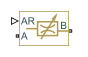# Variable Area Hydraulic Orifice

Hydraulic variable orifice created by cylindrical spool and sleeve

## Library

Hydraulic Elements

•## Description

The Variable Area Hydraulic Orifice block models a variable orifice created by a cylindrical sharp-edged spool and a variable-area slot in a sleeve. The area of the orifice is expected to be computed outside the block and imported via the AR physical signal connection. The Minimum area parameter specifies the minimum orifice area value. If the input signal falls below this level (for example, turns negative), the area is saturated to this value. The flow rate through the orifice is proportional to the orifice area and the pressure differential across the orifice.

The flow rate is determined according to the following equations:

`$q={C}_{D}\cdot A\sqrt{\frac{2}{\rho }}\cdot \frac{p}{{\left({p}^{2}+{p}_{cr}^{2}\right)}^{1/4}}$`
`$p={p}_{A}-{p}_{B}$`

where

 q Flow rate p Pressure differential pA, pB Gauge pressures at the block terminals CD Flow discharge coefficient A Orifice passage area ρ Fluid density pcr Minimum pressure for turbulent flow, when the block transitions from laminar to turbulent regime

The minimum pressure for turbulent flow, pcr, is calculated according to the laminar transition specification method:

• By pressure ratio — The transition from laminar to turbulent regime is defined by the following equations:

pcr = (pavg + patm)(1 – Blam)

pavg = (pA + pB)/2

where

 pavg Average pressure between the block terminals patm Atmospheric pressure, 101325 Pa Blam Pressure ratio at the transition between laminar and turbulent regimes (Laminar flow pressure ratio parameter value)
• By Reynolds number — The transition from laminar to turbulent regime is defined by the following equations:

`${p}_{cr}=\frac{\rho }{2}{\left(\frac{{\mathrm{Re}}_{cr}\cdot \nu }{{C}_{D}\cdot {D}_{H}}\right)}^{2}$`
`${D}_{H}=\sqrt{\frac{4A}{\pi }}$`

where

 DH Orifice hydraulic diameter ν Fluid kinematic viscosity Recr Critical Reynolds number (Critical Reynolds number parameter value)

The block positive direction is from port A to port B. This means that the flow rate is positive if it flows from A to B and the pressure differential is determined as $p={p}_{A}-{p}_{B}$.

## Variables

To set the priority and initial target values for the block variables prior to simulation, use the Initial Targets section in the block dialog box or Property Inspector. For more information, see Set Priority and Initial Target for Block Variables.

Nominal values provide a way to specify the expected magnitude of a variable in a model. Using system scaling based on nominal values increases the simulation robustness. Nominal values can come from different sources, one of which is the Nominal Values section in the block dialog box or Property Inspector. For more information, see Modify Nominal Values for a Block Variable.

## Basic Assumptions and Limitations

• Fluid inertia is not taken into account.

## Parameters

Flow discharge coefficient

Semi-empirical parameter for orifice capacity characterization. Its value depends on the geometrical properties of the orifice, and usually is provided in textbooks or manufacturer data sheets. The default value is `0.7`.

Minimum area

Leakage area of the completely closed orifice. If the input signal falls below this level (for example, turns negative), the area is saturated to this value. The parameter value must be greater than zero. The default value is `1e-12` m^2.

Laminar transition specification

Select how the block transitions between the laminar and turbulent regimes:

• `Pressure ratio` — The transition from laminar to turbulent regime is smooth and depends on the value of the Laminar flow pressure ratio parameter. This method provides better simulation robustness.

• `Reynolds number` — The transition from laminar to turbulent regime is assumed to take place when the Reynolds number reaches the value specified by the Critical Reynolds number parameter.

Laminar flow pressure ratio

Pressure ratio at which the flow transitions between laminar and turbulent regimes. The default value is `0.999`. This parameter is visible only if the Laminar transition specification parameter is set to ```Pressure ratio```.

Critical Reynolds number

The maximum Reynolds number for laminar flow. The value of the parameter depends on the orifice geometrical profile. You can find recommendations on the parameter value in hydraulics textbooks. The default value is `12`, which corresponds to a round orifice in thin material with sharp edges. This parameter is visible only if the Laminar transition specification parameter is set to `Reynolds number`.

## Global Parameters

Parameters determined by the type of working fluid:

• Fluid density

• Fluid kinematic viscosity

Use the Hydraulic Fluid (Simscape Fluids) block or the Custom Hydraulic Fluid block to specify the fluid properties.

## Ports

The block has the following ports:

`A`

Hydraulic conserving port associated with the orifice inlet.

`B`

Hydraulic conserving port associated with the orifice outlet.

`AR`

Physical signal port that provides the value of the orifice area.

## Version History

Introduced in R2009b